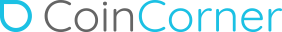# CoinCorner

CoinCorner are a leading gaming Bitcoin payment services provider. Established in 2014, their solution allows you to reach new markets while solving FX and chargeback issues. Players deposit in Bitcoin, and you receive daily settlement in GBP or EUR without exposure to the Bitcoin price. CoinCorner offers best-in-class user experience to both partners and players. Exchange and OTC services also available.

CoinCorner is available via CoinCorner in 46 countries.#### Available in:

• Country Transaction type
• Both
• Both
• Both
• Both
• Both
• Both
• Both
• Both
• Both
• Both
• Both
• Both
• Both
• Both
• Both
• Both
• Both
• Both
• Both
• Both
• Both
• Both
• Both
• Both
• Both
• Both
• Both
• Both
• Both
• Both
• Both
• Both
• Both
• Both
• Both
• Both
• Both
• Both
• Both
• Both
• Both
• Both
• Both
• Both
• Both
• Both
• Both
• Both
• Both
• Both
• Both
• Both
• Both
• Both
• Both
• Both
• Both
• Both
• Both
• Both
• Both
• Both
• Both
• Both
• Both
• Both
• Both
• Both
• Both
• Both
• Both
• Both
• Both
• Both
• Both
• Both
• Both
• Both
• Both
• Both
• Both
• Both
• Both
• Both
• Both
• Both
• Both
• Both
• Both
• Both
• Both
• Both
• Both
• Both
• Both
• Both
• Both
• Both
• Both
• Both
• Both
• Both
• Both
• Both
• Both
• Both
• Both
• Both
• Both
• Both
• Both
• Both
• Both
• Both
• Both
• Both
• Both
• Both
• Both
• Both
• Both
• Both
• Both
• Both
• Both
• Both
• Both
• Both
• Both
• Both
• Both
• Both
• Both
• Both
• Both
• Both
• Both
• Both
• Both
• Both
• Both
• Both
• Both
• Both
• Both
• Both
• Both
• Both
• Both
• Both
• Both
• Both
• Both
• Both
• Both
• Both
• Both
• Both
• Both
• Both
• Both
• Both
• Both
• Both
• Both
• Both
• Both
• Both
• Both
• Both
• Both
• Both
• Both
• Both
• Both
• Both
• Both
• Both
• Both
• Both
• Both
• Both
• Both
• Both
• Both
• Both
• Both
• Both
• Both
• Both
• Both
• Both
• Both
• Both
• Both
• Both
• Both
• Both
• Both
• Both
• Both
• Both
• Both
• Both
• Both
• Both
• Both
• Both
• Both
• Both
• Both
• Both
• Both
• Both
• Both
• Both
• Both
• Both
• Both
• Both
• Both
• Both
• Both